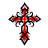# MomoAlphaTrigger (Day to Year) quote for ThinkorSwim#### wtf_dude

##### Active member
No idea if anybody would want this. Built it a year or 2 ago. Yea, some of the letter coding is out of order (I'm not a big coding guy and have severe concentration issues)
Feel free to play around with it.

Add this to your quote screen. It will average the return of the week, month, quarter and year and confirm that it's positive,
Then add the day and the week's returns to add more recent weight and see if the number remains positive,
Then see checks to see if it's beating SPX by more than 25bps,
Then checks to see if the RSI is over 50

If all tests check out, it will give you a green go signal and count the number of days since the signal turned green. Vice versa the tests fail.
Simple, but has helped me a lot with a quick glance for market winners

Code:
``````# Ultimate MomoAlphaTrigger (Day to Year) by WTF_Dude
# This script identifies days passed since time frame composites are +, alpha is over 25bps, RSI over 50
# if days passed is less than or equal to 5 then we assign light green/red color
# if days passed is greater than or equal to 5 then we assign dark green/red color
# More weight on day and week. Averages week, month, quarter, year.
# Only makes sure the composite is positive. No threshold minimum >0

def price = close;
def f = ((close - close) / close) * 100;
def a = ((close - close) / close) * 100;
def b = ((close - close) / close) * 100;
def c = ((close - close) / close) * 100;
def d = ((close - close) / close) * 100;
def e = ((a + b + c + d) / 4);
def h = f + a + e;

def ALPHA = AlphaJensen(index = "SPX") ;
def RSI1 = RSI(14);

# Identify bars where returns are up
def MOMO1up = e > 0;
def MOMO2up = h > 0;
def ALPHAup = ALPHA>.25;
def RSIup=RSI1>50;

#identify bars for 4up or 4down
def FourUP = MOMO1up and MOMO2up and ALPHAup and RSIup;
def FourDN = !MOMO1up and !MOMO2up and !ALPHAup and !RSIup;
#counter code
def FourUP_Count  = CompoundValue(1, if FourUP != FourUP then 1 else FourUP_Count + 1, 0);
def FourDN_Count  = CompoundValue(1, if FourDN != FourDN then -1 else FourDN_Count + 1 * -1, 0);
def data = if FourUP then FourUP_Count  else if FourDN then FourDN_Count else Double.NaN;
#Assign Back Ground Color

# HINT: Change CreateColor(237,237,237) to Color.BLACK or to any other color that matches your background

AssignBackgroundColor(if FourUP then (if data <= 3 then Color.GREEN else Color.DARK_GREEN ) else if FourDN then (if data >= -3 then Color.RED else Color.DARK_RED) else Color.BLACK );

AddLabel(!IsNaN(data), data, if FourUP then (if data <= 3 then Color.BLACK else Color.WHITE) else if FourDN then (if data >= -3 then Color.WHITE else CreateColor(237, 237, 237)) else Color.BLACK);

AddLabel(IsNaN(data), " ", CreateColor(237, 237, 237));``````Double Bollinger Bands (DBB) for ThinkorSwim Indicators 8